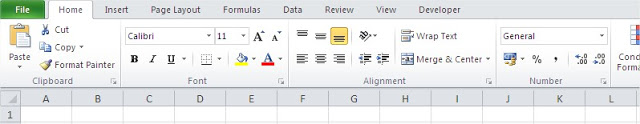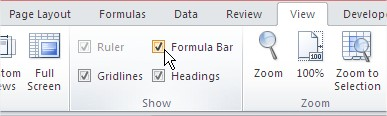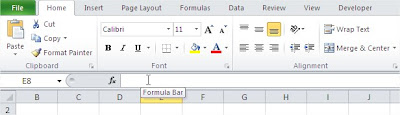#### How to Fix Missing Formula Bar Issue

You can’t find a formula bar? In this lesson you can learn how to fix it.

## How to show Excel formula bar?

Sometimes there is a simple problem in Excel. You can’t choose your formula, because the formula bar is missing.If the formula bar is missing in Excel, you can easily show it back by following these steps:

In the Show group, check the box next to Formula Bar.The formula bar should now reappear.## How to Always Show the Formula Bar in Excel

If you find yourself frequently needing to show the formula bar, you can set Excel to always show it. To do this, follow these steps:

1. Click on the File tab.
2. Click on the Options button.
3. In the Excel Options dialog box, click on the Advanced tab.
4. In the Display section, scroll down to the Show group and check the box next to Always show formula bar.

The formula bar will now always be visible, even when you are not in edit mode.

See also  How to resolve Excel stopped interacting with windows and was closed issue